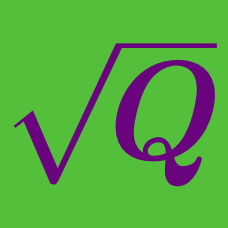Algebra

Radical Expressions and Equations: Level 2 Challenges

$\large \sqrt { -4 } \times \sqrt { -9 } = \, ?$

$\large \dfrac{\sqrt{50}+7}{\sqrt{50}-7}+\dfrac{\sqrt{50}-7}{\sqrt{50}+7} = \ ?$

$\Huge \sqrt{\color{#D61F06}9^{\color{#20A900}{16} \color{#3D99F6}{x^2}}}$

Which of the following is equal to the above expression?

$\Large{ \sqrt{3+2\sqrt2} - \sqrt{3-2\sqrt2} = \ ?}$

Which is greater?

$\Large\sqrt{ \color{#D61F06}{8}} - \sqrt{ \color{#D61F06}{7}} \ \ \ \ \ \text{OR} \ \ \ \ \ \sqrt{ \color{#3D99F6}{6}} - \sqrt{ \color{#3D99F6}{5}}$

×